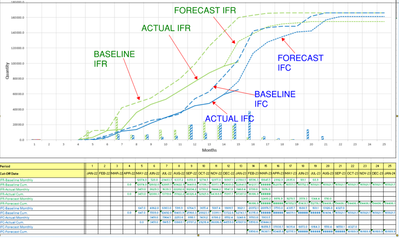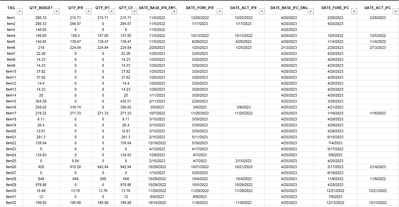cancel
Showing results for
Did you mean:Regular Visitor

## BASELINE ACTUAL AND FORECAST CURVE BY QTY AND DATES

hello

I'm trying to create a set of curve (baseline-forecast-actual) as shown below.curves are base on the following DB, corresponding with a set of items to be issued in two steps (IFR and IFC)BASELINE IFR CURVE: calculated based of QTY_BUDGET + DATE_BASE_IFR_ENG

ACTUAL IFR CURVE: calculated based of QTY_IFR + DATE_ACT_IFR

FORECAST IFR CURVE: calculated based of QTY_CE + DATE_FORE_IFR

BASELINE IFC CURVE: calculated based of QTY_BUDGET + DATE_BASE_IFC_ENG

ACTUAL IFC CURVE: calculated based of QTY_IFC + DATE_ACT_IFC

FORECAST IFC CURVE: calculated based of QTY_CE + DATE_FORE_IFC

The range of date shall be from JAN-22 TO JAN-24

any support on this is highly appreciated.

thanks

0 REPLIES 0